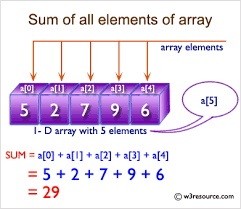# C Program To Find Sum Of All Array Elements | 4 Simple Ways

C Program to find the sum of all array elements – In this article, we will detail in on all the ways to find the sum of all array elements in C programming.

Suitable examples and sample programs have also been added so that you can understand the whole thing very clearly. The compiler has also been added with which you can execute it yourself.

The means used in this distinct piece are as follows:

• Using Standard Method
• Using Function
• Using Recursion

An array in the C Programming language can be termed as a sequence of orderly arrangements of things of a single type.

This is what an array looks like:Source: w3resource.com

As you can see, 5 elements are present in this array. Each element has been given the location a to a.

Thus, adding up all the elements would give us:

Sum = 5 + 2 + 7 + 9 + 6 = 29.

Thus, the multitude of methods used to calculate the sum of all the elements of an array is as follows:

## Using Standard Method

1. Read the array size and store it in the variable n.

2) Scanf function reads the entered element and store the element  in to the array as a[i] using for(i=0;i<n;i++) loop.

3) sum=0, add each element of the array to the sum value using for loop with the structure for(i=0; i<n;i++) as sum=sum+a[i].

4) After all iterations of for loop, we will get the sum of all array elements.

Output:

### Using Function

1. The function sumofarray() is the user defined function which calculates the sum of all array elements of an array.

2) The main() function calls the sumofarray() function by passing an array, size of an array.

3)The function sumofarray(int a[], int n) adds the each element of the array to the sum value using for loop with the structure for(i=0;i<n;i++) and returns the sum value to main() function.

4) The main() function prints the sum value which contains the sum of all elements of the given array.

Output:

#### Using Recursion

1. The main function calls the sumofarray() function by passing an array, size of the array, i value.

2) The function sumofarray() calculates the sum of all elements of the array by calling itself until the condition i<n becomes false.

3) The sumofarray() returns the sum value to main() function,then main() function prints the sum value.

Here sumofarray() function is the recursive function which calls itself until the condition becomes false.

Output:
x

## C Program To Check If Alphabet, Digit or Special Character | C Programs

C program to check for an alphabet, digit or special character – In this article, ...